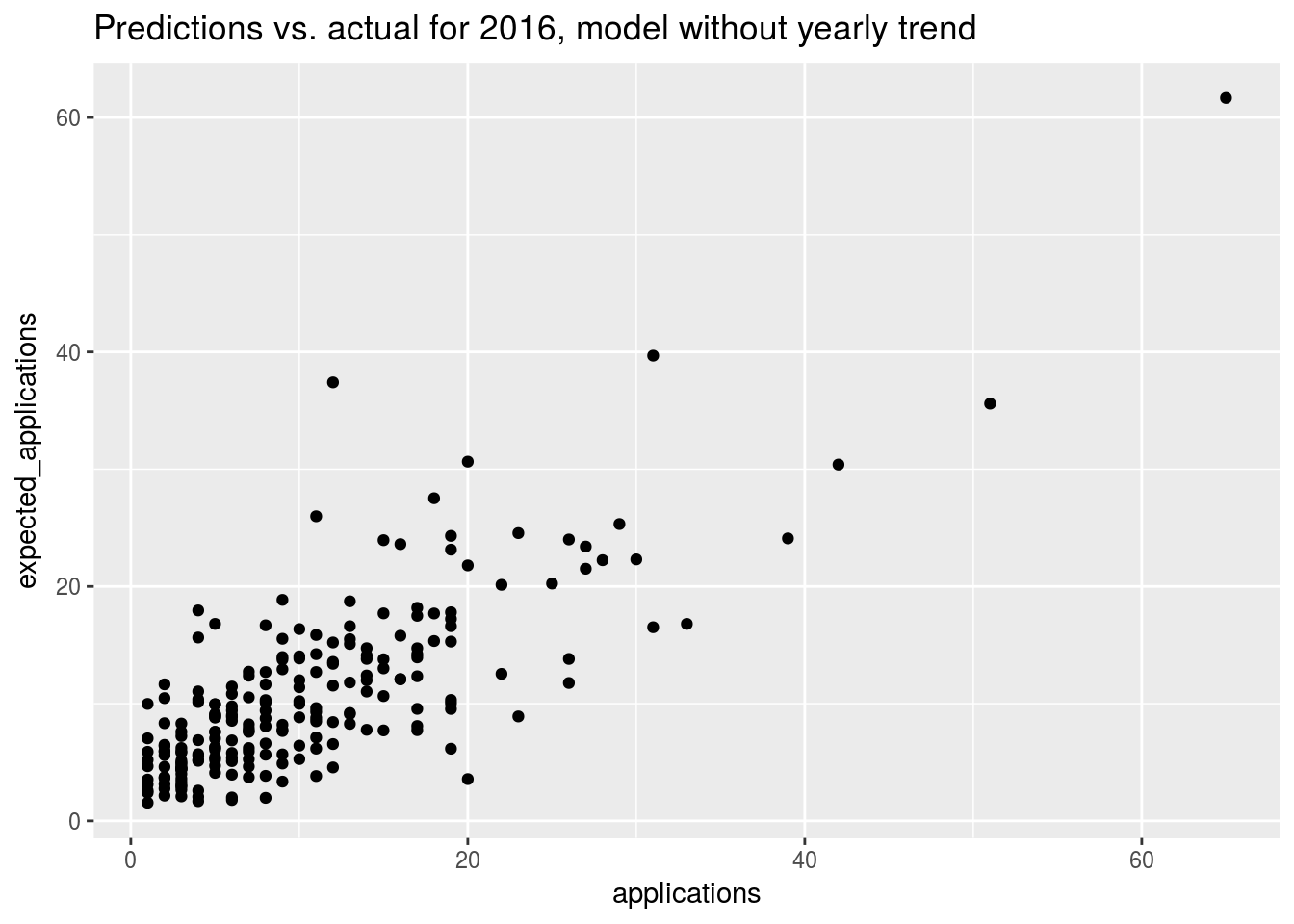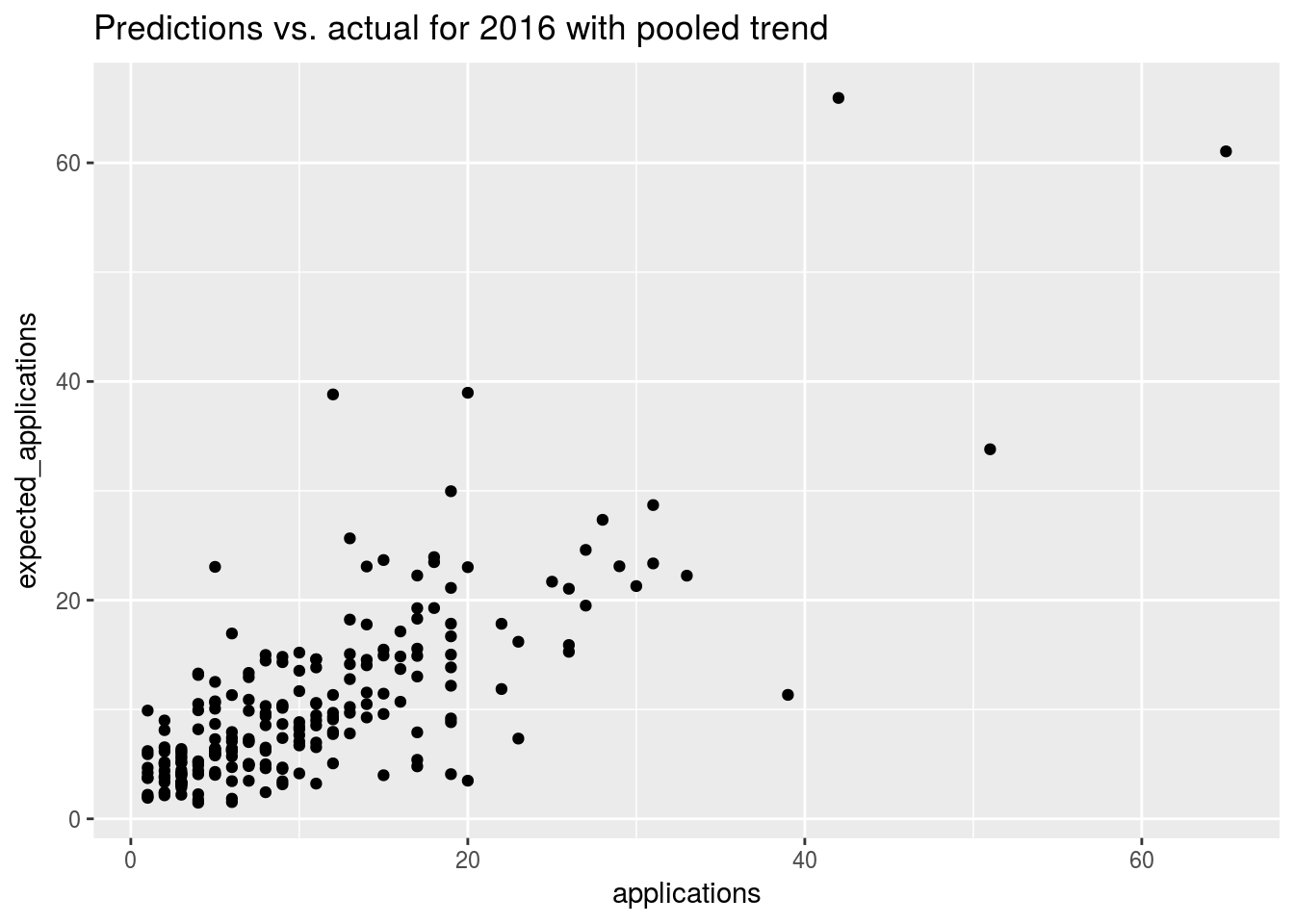A quick comparison of predictions and AIC values for school admissions
library(dplyr)
##
## Attaching package: 'dplyr'
## The following objects are masked from 'package:stats':
##
##     filter, lag
## The following objects are masked from 'package:base':
##
##     intersect, setdiff, setequal, union
library(ggplot2)

schools <- read.csv("./schools.csv")
• AIC comparison shows that the model with a trend looks better, and there is definitely variation between the schools
• The multilevel models don’t help here
library(lme4)
## Loading required package: Matrix
base <- glm(cbind(applications, population) ~ 1, data = schools, family = "binomial")
fit.nml <- glm(cbind(applications, population) ~ 1 + school, data = schools, family = "binomial")
fit.nml.wtrend <- glm(cbind(applications, population) ~ 1 + school * year, data = schools, family = "binomial")
fit.ml.wtrend <- glmer(cbind(applications, population) ~ 1 + scale(year) + (1 + scale(year)| school), data = schools, family = "binomial")
fit.ml <- glmer(cbind(applications, population) ~ 1 + (1| school), data = schools, family = "binomial")

AIC(base, fit.nml, fit.nml.wtrend, fit.ml.wtrend, fit.ml)
##                 df       AIC
## base             1 13912.785
## fit.nml        224  6474.879
## fit.nml.wtrend 448  6375.948
## fit.ml.wtrend    5  6887.382
## fit.ml           2  7017.367

## Gaussian model without trend

fit <- glm(applications ~ 1 + school, data = schools %>% filter(year < 2016), family = "gaussian")

preds <- schools %>% mutate(
expected_applications = predict(fit, schools)
) %>% filter(year == 2016)

mean((preds$expected_applications - preds$applications) ** 2)
##  29.71987

## Gaussian model with trend, multilevel

fit <- glmer(applications ~ 1 + scale(year) + (1 + scale(year) | school), data = schools %>% filter(year < 2016), family = "gaussian")
## Warning in glmer(applications ~ 1 + scale(year) + (1 + scale(year) |
## school), : calling glmer() with family=gaussian (identity link) as a
## shortcut to lmer() is deprecated; please call lmer() directly
preds <- schools %>% mutate(
expected_applications = predict(fit, schools)
) %>% filter(year == 2016)

mean((preds$expected_applications - preds$applications) ** 2)
##  29.51741

## Binomial model

fit <- glm(cbind(applications, population) ~ 1 + school, data = schools %>% filter(year < 2016), family = "binomial")

preds <- schools %>% mutate(
predicted_proportion = predict(fit, schools, type = "response"),
expected_applications = population * predicted_proportion
) %>% filter(year == 2016)

mean((preds$expected_applications - preds$applications) ** 2)
##  30.97042
ggplot(preds, aes(x = applications, y = expected_applications)) + geom_point() + labs(
title = "Predictions vs. actual for 2016, model without yearly trend"
)• The model without the trend has better predictions. A trend based on 3 years is maybe a bit too noisy.

## Multilevel trend estimation

model <- glmer(cbind(applications, population) ~ 1 + scale(year) + (1 + scale(year) | school),
data = schools %>% filter(year < 2016),
family = "binomial")

preds <- schools %>% mutate(
predicted_proportion = predict(model, schools, type = "response"),
expected_applications = population * predicted_proportion
) %>% filter(year == 2016)

mean((preds$expected_applications - preds$applications) ** 2)
##  35.79241
ggplot(preds, aes(x = applications, y = expected_applications)) + geom_point() + labs(
title = "Predictions vs. actual for 2016 with pooled trend"
)